# Hybridization

## Hybridization - Types and Examples

Hybridization is a concept used in organic chemistry to explain the chemical bonding in cases where the valence bond theory does not provide satisfactory clarification. This theory is especially useful to explain the covalent bonds in organic molecules.
Basically, hybridization is intermixing of atomic orbitals of different shapes and nearly the same energy to give the same number of hybrid orbitals of the same shape, equal energy and orientation such that there is minimum repulsion between these hybridized orbitals.

Explanation:

Consider an example of the simplest hydrocarbon molecular Methane. CH4.

According to experimental observations, Methane molecule has 4 identical C-H bonds with equal length and equal bond energy. All the four hydrogen atoms are arranged in a manner such that the four hydrogen atoms form corners of a regular tetrahedron.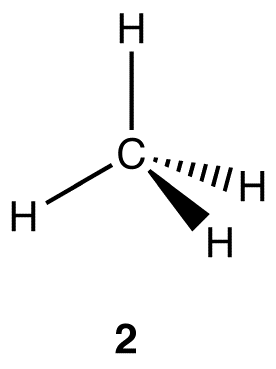Based on the valence theory, a covalent bond is formed between two atoms in a molecule when there is an overlapping of half-filled atomic orbitals containing unpaired electrons. In the case of the methane molecule, we first write down the electronic configuration of each atom - C and H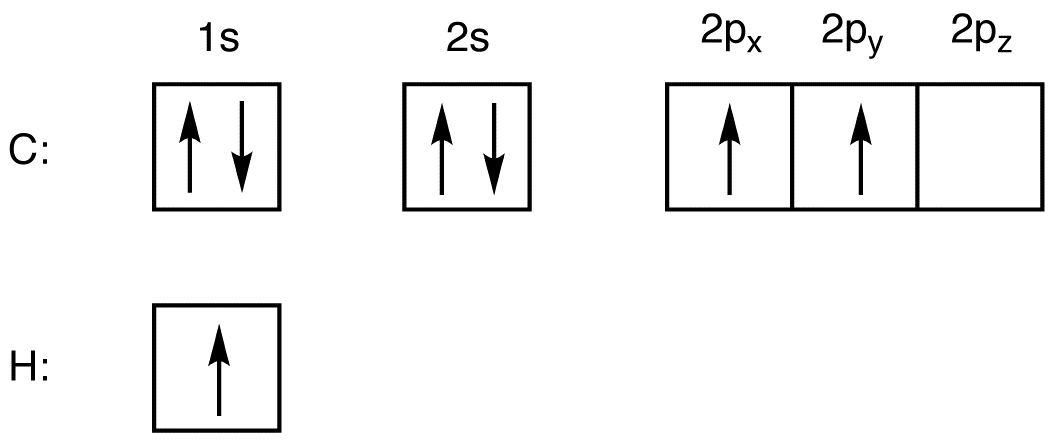Each carbon atom has two unpaired electrons (in the 2px and 2py orbitals). Based on the valence theory, only two hydrogen molecules could be paired to the two unpaired electrons of the carbon atom and there will be a formation of only 2 C-H bonds in the molecule. This will lead to an incomplete octet in the 2nd orbital of the carbon molecule (2pz orbital is unfilled) and so the molecule should be unstable. However, we see that actually the methane molecule is extremely stable in nature and has 4 C-H bonds and not two. Thus, the valence theory doesn’t explain the covalent bond of the methane molecule.

Hybridization concept explains the formation of identical 4 C-H bonds and the tetrahedral shape of the molecule.

According to this concept, when a carbon atom reacts with a hydrogen atom, the electrons in the carbon atom initially go into an excited state as shown here: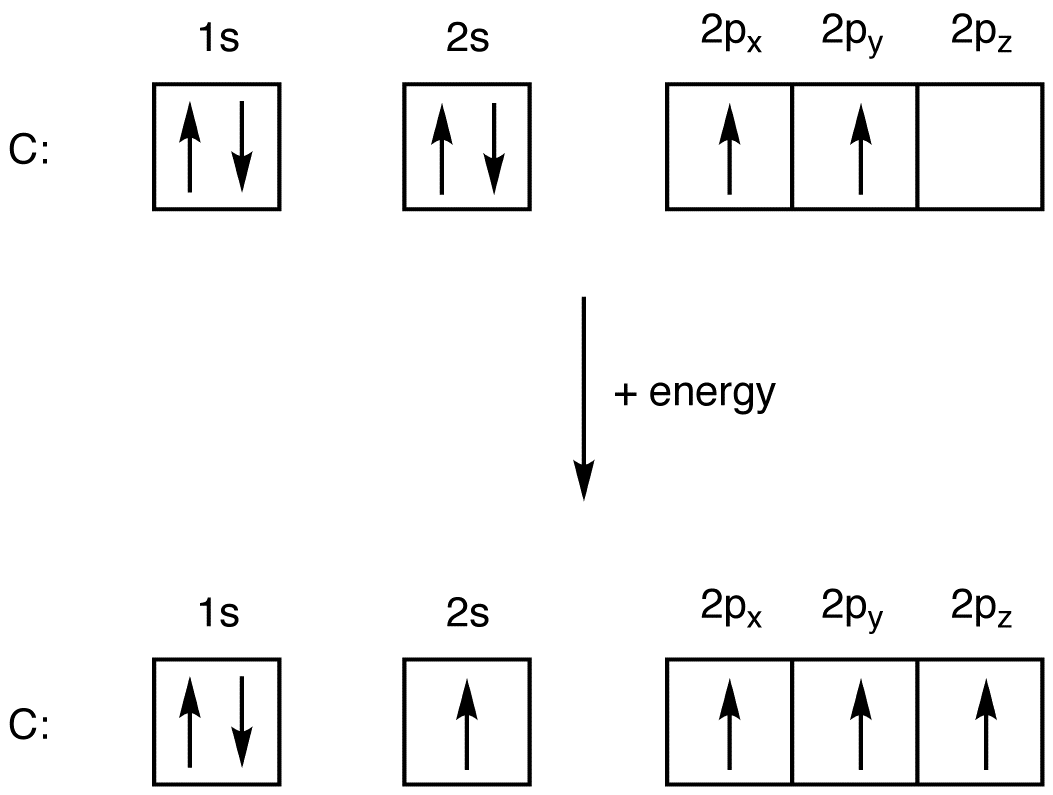Post excitation, hybridization can be imagined as the process where these 4 excited s and the p orbitals combine together to give a homogenous mixture and divide themselves into 4 identical orbitals having identical energy. These new orbitals have been termed as hybridized orbitals. Since there one s orbital and 3 p orbitals have combined to form the hybrid orbital, the hybridized orbitals are called as sp3 orbitals. The energy of these hybrid orbitals lie in between the energy levels of the s and the p orbitals as shown here:Each sp3 hybrid orbitals has one unpaired electron. Since these 4 sp3 orbitals are identical in terms of energy, there is a tendency amongst these electrons to repel each other. To minimize the repulsion between electrons, the sp3hybridized orbitals arrange themselves around the carbon nucleus in a tetrahedral arrangement. The resulting carbon atom is termed as sp3 hybridized carbon atom.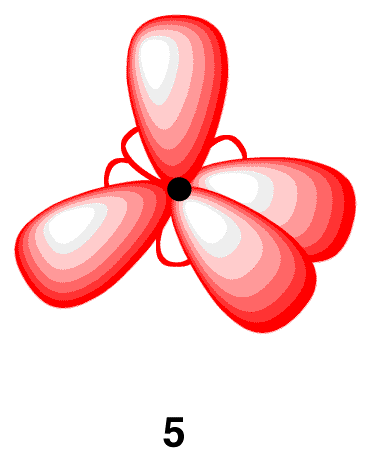Overlap of each of the 4 sp3orbitals of the hybridized carbon atom with the s orbital of the hydrogen atoms leads to the formation of methane molecule. The methane molecule can be shown as: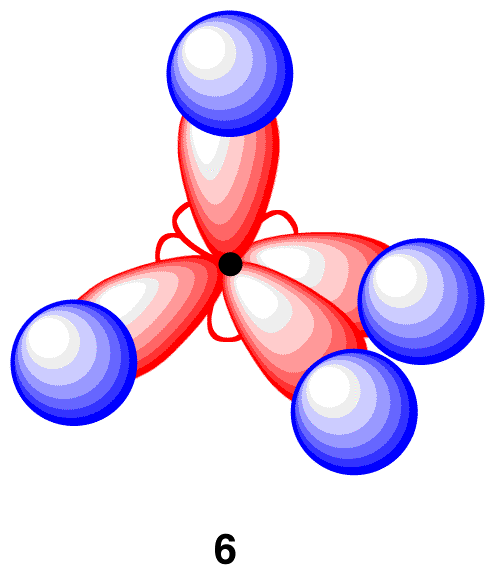It can be seen from the above that there are 4 identical sp3-s overlap forming 4 identical C-H bonds which is consistent with the observations. Moreover, since these sp3 orbitals are oriented in the form of tetrahedron, the geometry of the methane molecule is tetrahedral.

Thus, adding the concept of hybridization to the valence theory helps to understand the bonding in the methane molecule.
The above example of methane had sp3 hybridization formed because of hybridization of 1 s and 3 p orbitals of the carbon atom. There are other types of hybridization when there are hybrid orbitals between 2 p orbitals and 1 s orbital called sp2 hybridization. In case, there are hybrid orbitals between 1 s and 1 p orbitals, it is called sp hybridization.

Let us consider the case of sp2 hybridization. The structure of ethylene can be explained using the concept of sp2 hybridization. The structure of the ethylene molecule observed is as: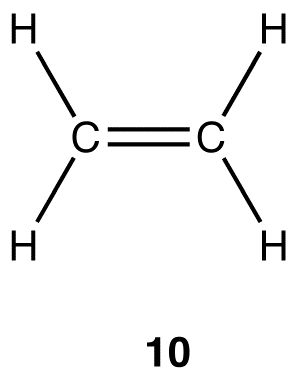Experimentally, the four carbon-hydrogen bonds in the ethylene molecule are identical and the geometry at each carbon atom in the ethylene molecule is planar trigonal.

Since the carbon atom has only 2 unpaired electrons, the valence bond theory cannot explain the formation of 4 bonds by each of the carbon atoms. Hence, we have to consider the excited state of both the carbon atoms in order that each carbon atoms form 4 bonds.

We first consider the two carbon atoms and the double bond between them.

For each of the excited carbon atoms, the one 2s orbital and two 2p orbitals (of the three 2p orbitals) form hybridization resulting in 3 hybrid orbitals called sp2 orbitals. (1 s and 2 p orbitals). These 3 sp2 orbitals try to be as distant from each other as possible and hence form a planar triagonal structure. The third 2p orbital in each of the carbon atom does not participate in hybridization and remains as 2p orbital.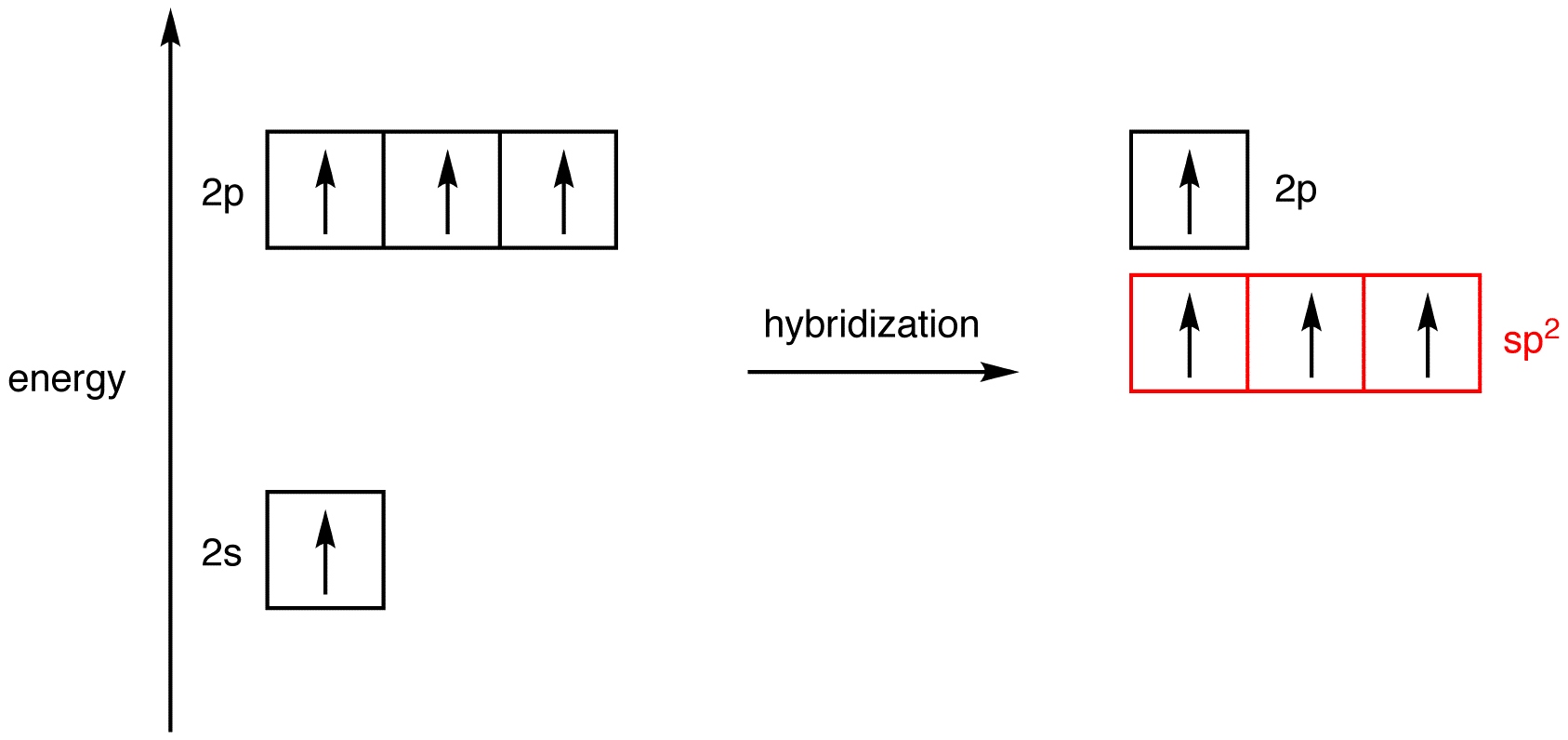Each of the three sp2 hybrid orbital and the unhybrid 2p orbital has 1 unpaired electron. To minimize repulsion of this unhybrid 2p orbital with the 3 sp2 orbitals, 2p orbital stands perpendicular to each of the sp2 hybrid orbitals. Hence, post-hybridization, the sp2 hybridized carbon atom looks as: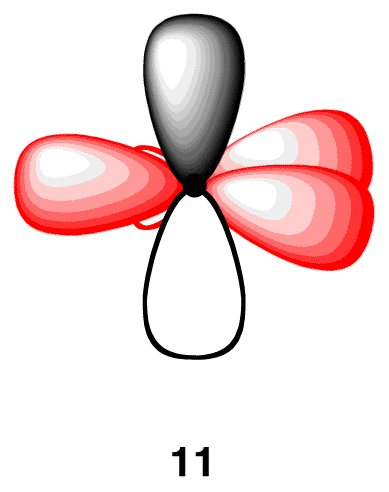Each carbon atom in the ethylene molecule is bonded to two hydrogen atoms. Thus, overlap two sp2-hybridized orbitals with the 1s orbitals of two hydrogen atoms Also, the covalent C-C bond forms by overlapping of sp2 orbitals of the two carbon atoms as: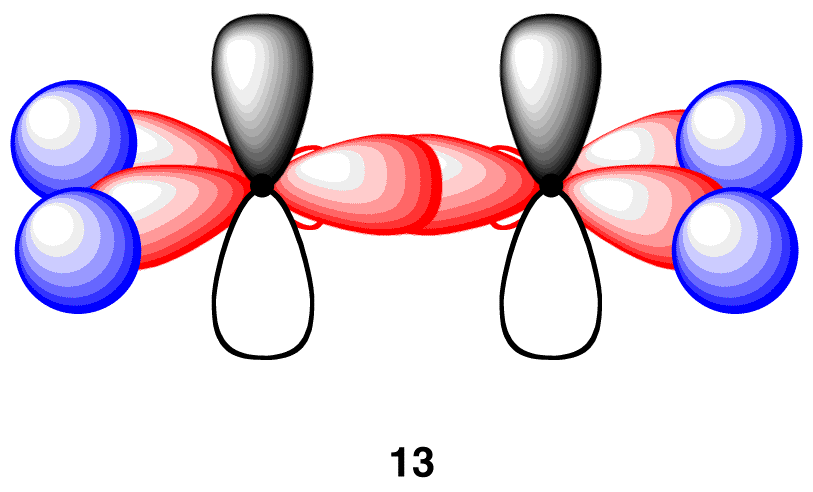The two 2p orbitals of the carbon atoms overlap laterally form a weak bond called a pi bond.

Thus, the ethylene molecule is said to have sp2-s bonds (4 C-H bonds) , one sp2-sp2 bond (C-C bond) and one p-p pi bond (C-C bond).

Thus, the sp2 hybridization theory explains the double bond, the trigonal planar structure in ethylene molecule.
Similarly, for a triple bond formation, like for that of acetylene molecule, there is sp hybridization between 1 s and 1 p orbital of the carbon atom.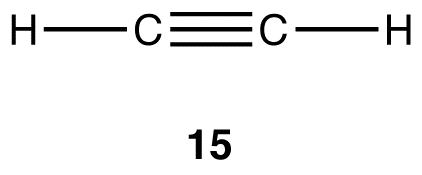Here, there are 2 C-H bonds and a triple C-C bond.

In each of the excited carbon atom, one 2s and one 2p orbital form hybrid molecules called sp hybrid orbitals and the unhybrid two 2p orbitals do not participate in hybridization. Because there are electron molecules in each of the orbitals, they tend to repel each other and the 2sporbitals form a linear arrangement. The unhybrid 2p orbitals 2p orbital position themselves as far away as possible from each sp-hybridized orbital when perpendicular to each sp-hybridized orbital. So the resulting sp hybrid carbon atom looks like: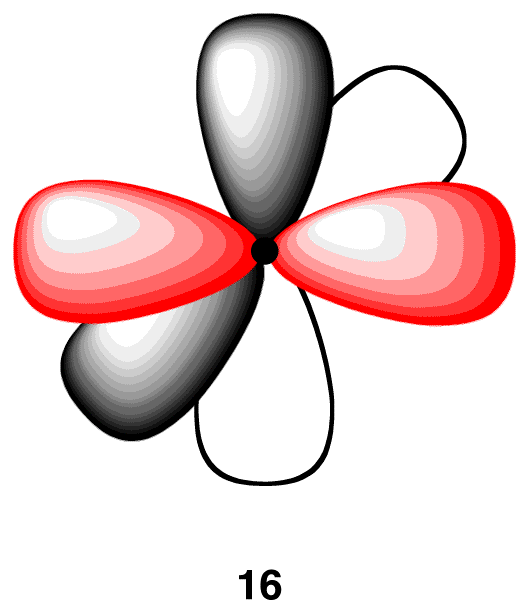The s orbitals of the hydrogen atom overlap with one sp hybrid orbital of each of the carbon atom forming the 2 C-H bonds. The C-C covalent bond is formed by overlapping between the sp-sp orbitals of the two hybrid carbon atoms. In order to complete octet, the two unhybrid 2p orbitals of each of the carbon atom overlap laterally forming 2 pi bonds as shown: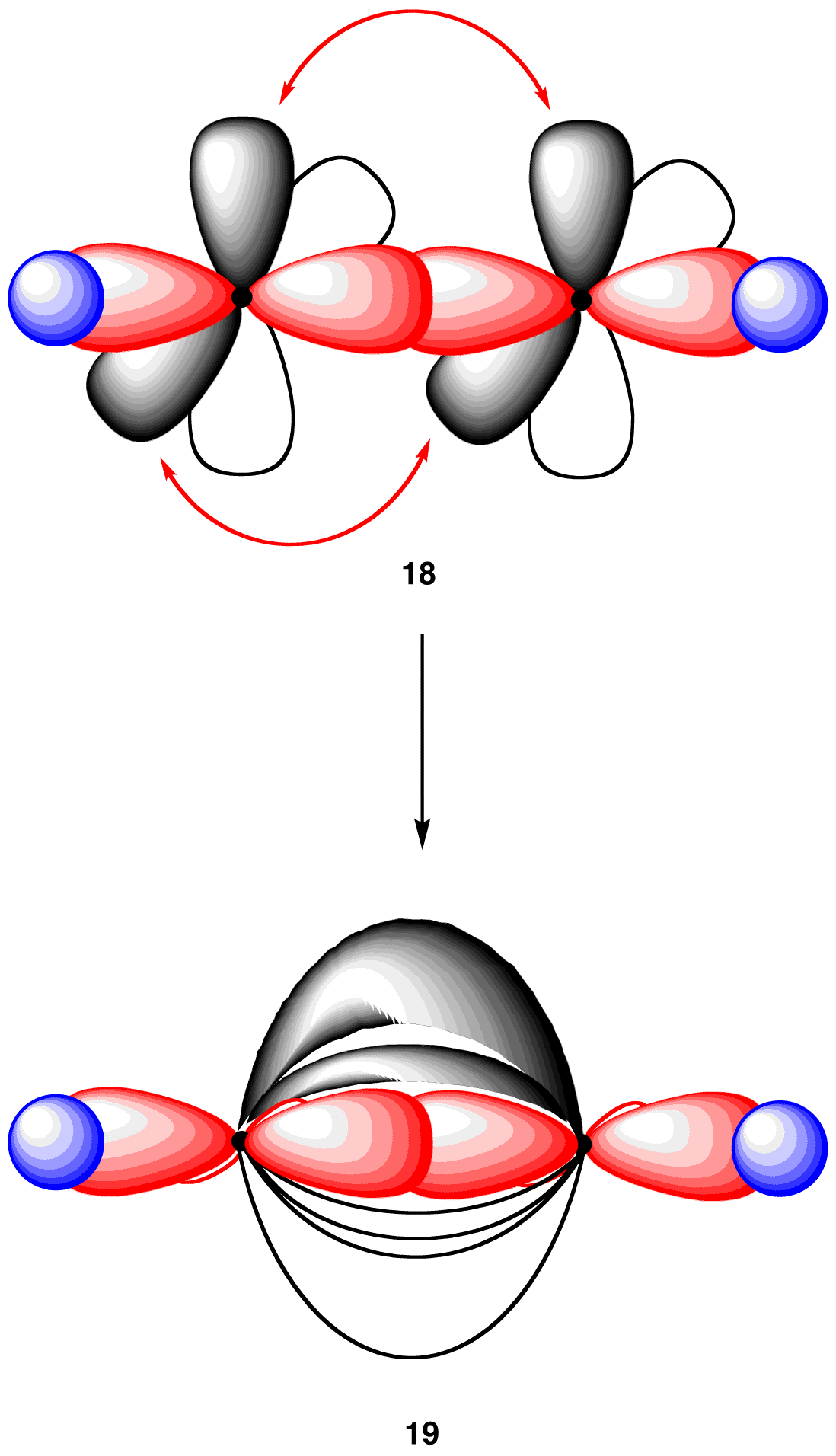Thus, sp hybridization explains the triple bond in acetylene molecule and the linear structure as well.

Thus, hybridization as a concept helps explain the molecular structure and shapes of the molecules. The following table summarizes the shapes of the molecules:

 Type of hybridization Shape Number of orbitals participating in hybridization sp3 Tetrahedral 4 (1s + 3p) sp2 Planar triganol 3(1s + 2p) sp Linear 2(1s + 1p)